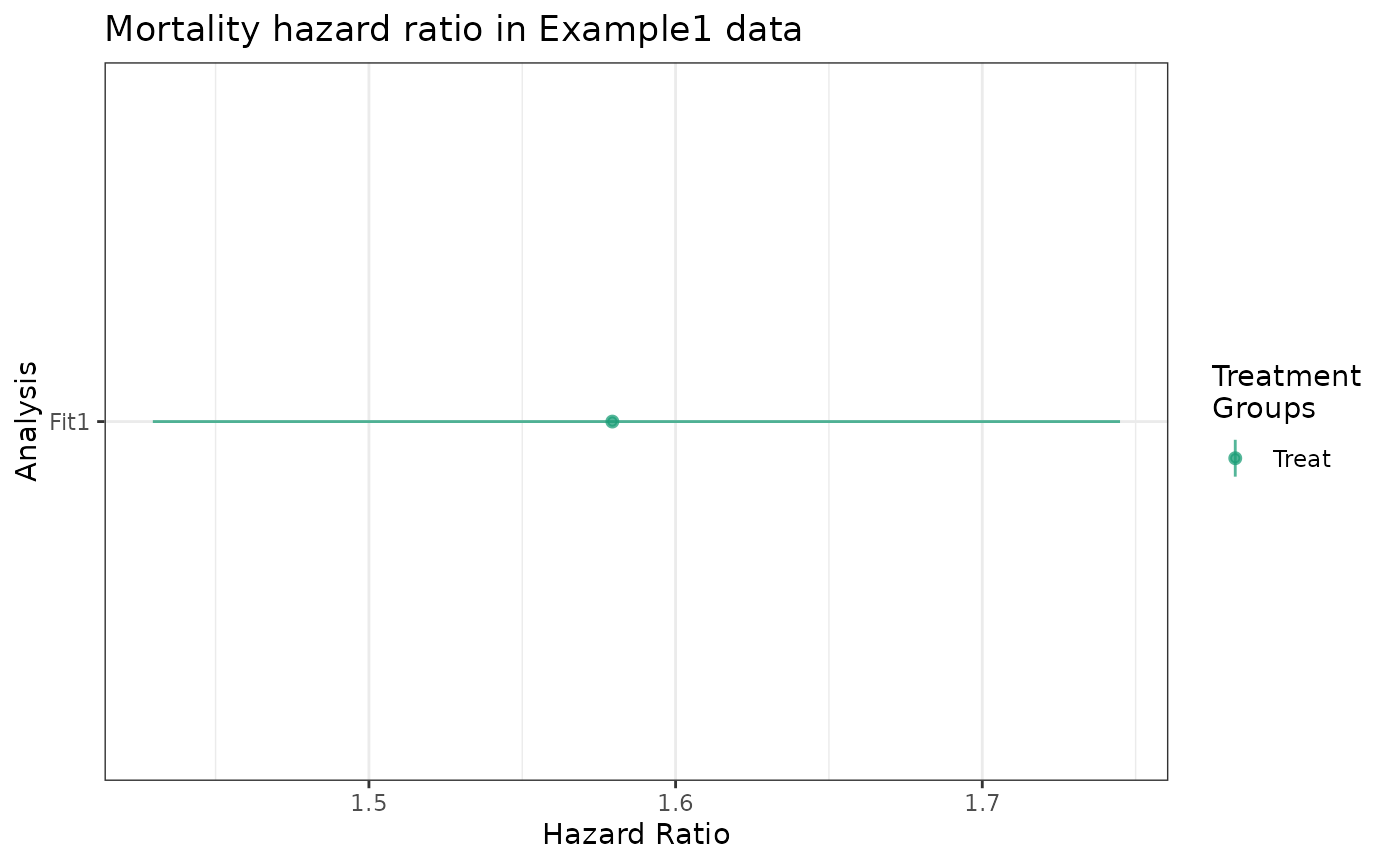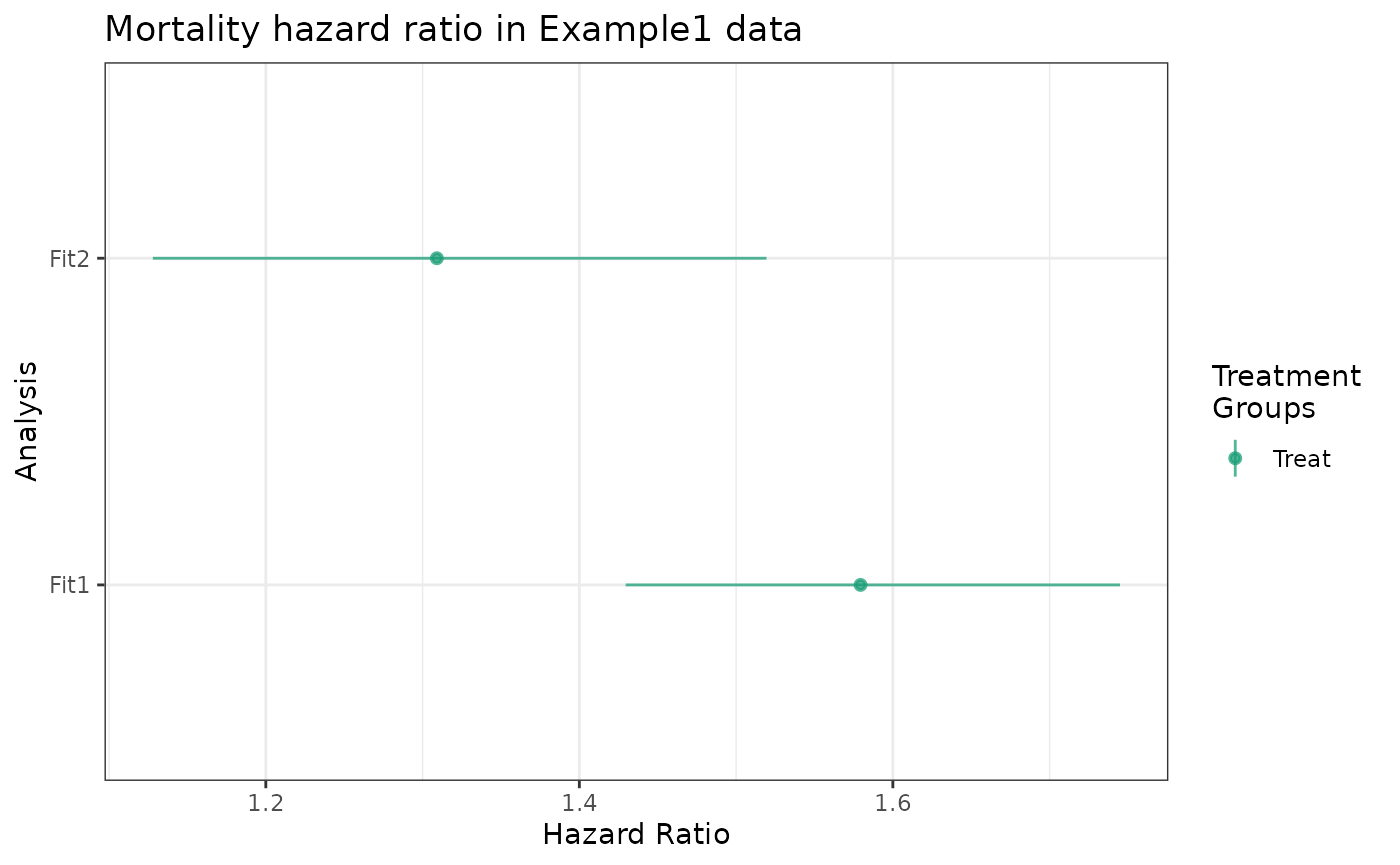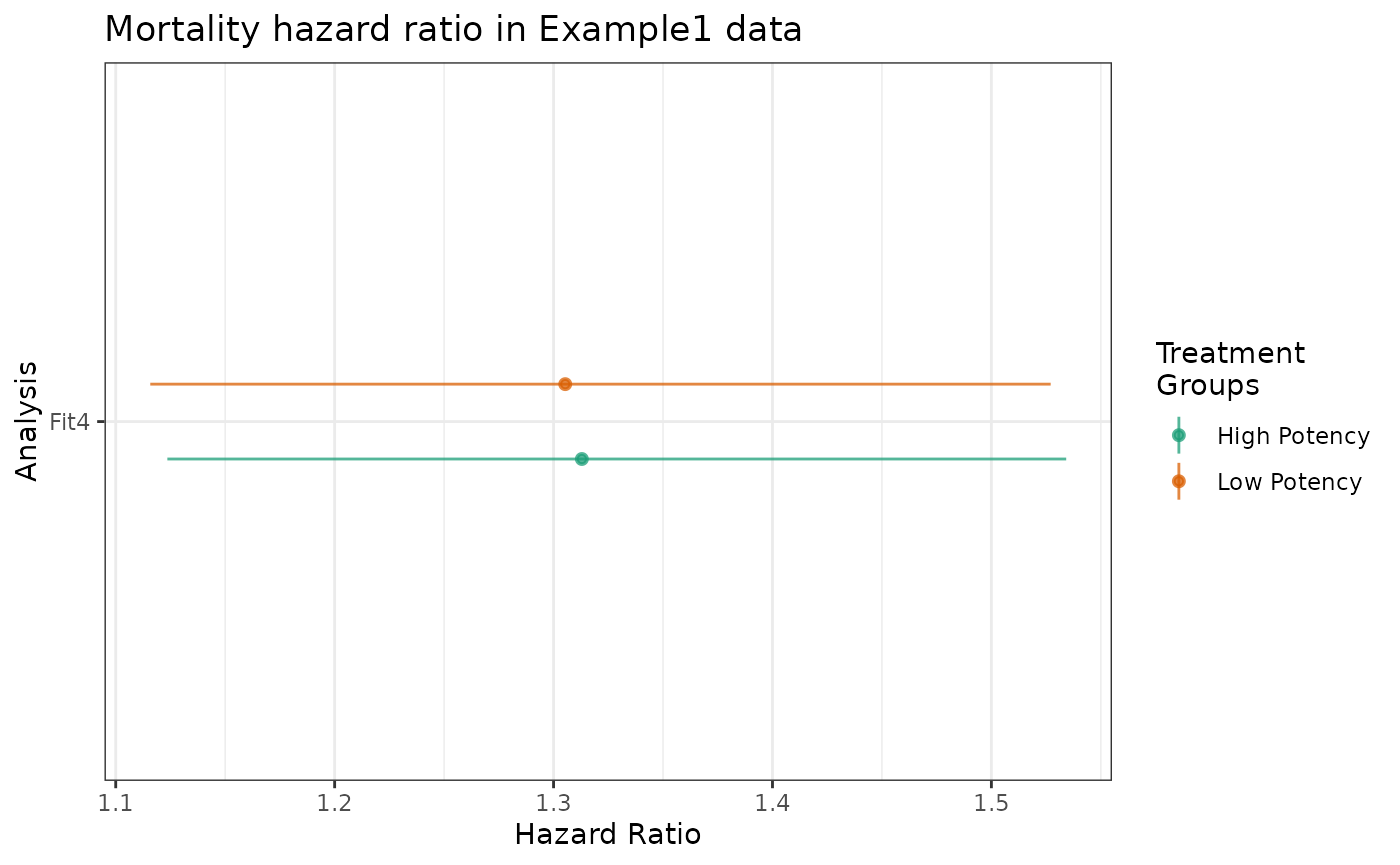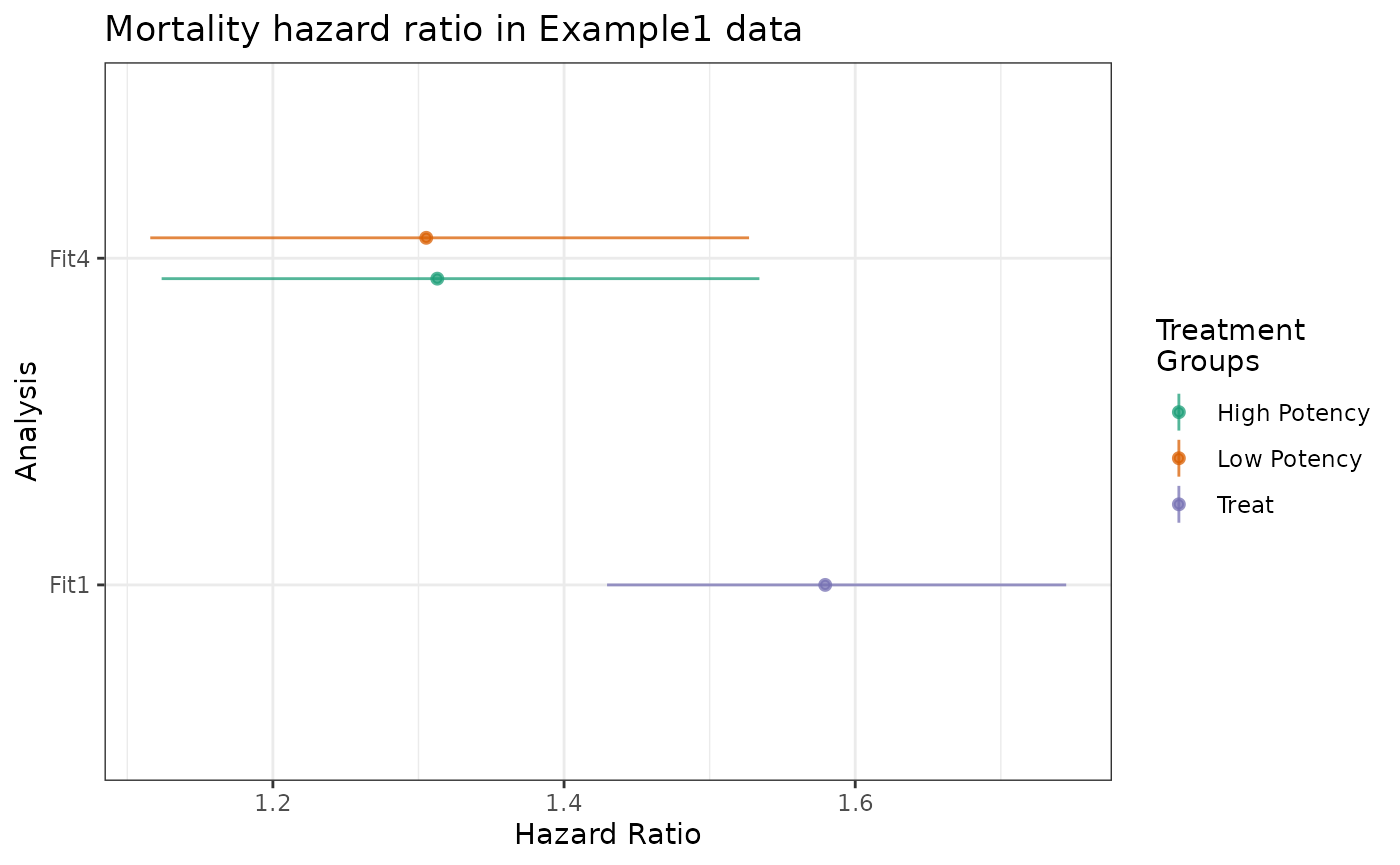## Overview

This vignette describes the estimate_ipwhr function, providing details of how hazard ratios are computed, the theoretical background for the implementation, and some examples extending those found in the causalRisk manual. estimate_ipwhr and methods for its resulting object hew as closely as possible to the API for estimate_ipwrisk.

estimate_ipwhr fits a weighted Cox model with the treatment variable as its only regressor, to estimate the causal effect of the treatment on the outcome, presented as hazard ratios relative to the reference group. In the case of a binary treatment, the procedure maximizes the partial likelihood

$L(\beta) = \prod_{i{:} \Delta_{i} = 1} {% \left[\frac{\exp(\beta X_i)}{\Sigma_{j \in R(t_{i})} \hat{w}_{j} \exp(\beta X_j)}\right] }^{\hat{w}_{i}},$

where $$\Delta_{i}$$ is an indicator of whether an event was observed before censoring for subject $$i$$, $$X_i$$ is the treatment indicator for subject $$i$$, $$\beta$$ is the regression coefficient corresponding to the log hazard ratio between the treatment groups, $$t_{i}$$ is the time at which an event occurred for subject $$i$$, $$R(t_{i})$$ is the risk set at time $$t_{i}$$, and $$\hat{w}_{i}$$ is a weight for subject $$i$$  (note that a slight modification of the likelihood is needed in the presence of tied event times).

In models without missingness, weights are inverse probabilities of treatment. When the model includes missingness, weights are the product of inverse probabilities of treatment and missingness. estimate_ipwhr does not currently support inverse probability of censoring weights.

### Model assumptions

The estimators of causal effects requires special assumptions. We focus on the core set of assumptions frequently used to support this class of semiparametric estimators: consistency, no inteference, no unmeasured confounders, positivity, no measurement error on exposures, confounders, or treatment, missing data are stratified missing completely at random, and all statistical models are correctly specified. Additonal details on these assumptions can be found in the causalRisk manual’s technical appendix.

In addition to those general assumptions, we make the proportional hazards assumption that the survival curves for the treatment groups have hazard functions that are proportional over time.

Since estimate_ipwhr does not currently support inverse probability of censoring weights, we assume that censoring is noninformative, that is the censoring hazard is independent of survival time conditional on the treatment and being at risk.

Finally, estimate_ipwhr does not currently support competing risks, so we assume there are no competing risks.

### Confidence intervals

The confidence intervals are computed using the robust standard errors produced by the Cox PH model. For IP weighted models with other types of outcomes like mean differences or differences in proportions, the robust standard errors have been shown to be conservative . We have not been able to find in the literature a reference that unambiguously proves that the robust standard errors are conservative for the hazard ratios estimated by an IP weighted Cox PH model.  states that the robust standard errors are conservative for the Cox PH model when there is no censoring. They also claim it holds when using a weighted pooled logistic regression model instead of a weighted Cox PH model, and they claim pooled logistic regression model is “essentially equivalent” to the Cox PH model.  indirectly claims that the robust standard errors are conservative for the IP weighted Cox PH model, but the citation they reference in support of this claim is in the context of IP weighted models for a continuous outcome.  includes a simulation study that provides empirical support for the claim that the robust standard errors provide conservative variance estimation for the hazard ratios estimated by an IP weighted Cox PH model.

## Basic usage

This example extends the section Summarizing cumulative risk by treatment group from the causalRisk manual, providing a hazard ratio for the treatment model instead of cumulative risk curves.

As described in the aforementioned section, the model can be defined as follows.

models <- specify_models(identify_treatment(Statin),
identify_outcome(Death))

But now, instead of calling estimate_ipwrisk to produce cumulative risk estimates, we use estimate_ipwhr to estimate hazard ratios. The object fit1_hr has class hr.

fit1_hr <- estimate_ipwhr(example1,
models,
tau = 24,
labels = "Fit1")

The plot method is identical to the forest_plot method, returning an object of class “ggplot” giving the estimated hazard ratio and, by default, an $$0.05$$ -level confidence interval.

Ratios relative to the reference group are provided for all treatment groups. In this example, the treatment variable Statin has only two levels: “Treat” and “Control”.

plot(fit1_hr) +
ggtitle("Mortality hazard ratio in Example1 data")The make_table2 method when applied to estimate_ipwhr objects behaves analogously to it’s application to estimate_ipwrisk objects, producing summaries on the hazard ratio scale rather than the risk difference or relative ratio scales.

make_table2(fit1_hr)

Both the plot and make_table2 methods allow for specifying different confidence levels via the alpha argument.

make_table2(fit1_hr, alpha = 0.01)

## Estimating hazard ratios with IPT weights

This example demonstrates the usage of estimate_ipwhr in which an IPTW model is used to produce per-subject weights.

estimate_ipwhr fits the treatment model with estimate_ipwrisk to the treatment weights, whose product is passed a the weights argument to survival::coxph. See the Overview section for a more technical description of that procedure.

models <- specify_models(identify_treatment(Statin, formula = ~DxRisk),
identify_outcome(Death))

Here, we use the tau = 24 argument to specify the maximum follow-up time during this study.

fit2_hr <- estimate_ipwhr(example1,
models,
tau = 24,
labels = "Fit2")

As with the cumrisk methods, hr class plot and make_table2 methods support passing multiple objects resulting from estimate_ipwhr. It is important to provide unique labels to each object before passing them into plot or make_table2.

plot(fit1_hr, fit2_hr) +
ggtitle("Mortality hazard ratio in Example1 data")make_table2(fit1_hr, fit2_hr)

### Changing the reference group

The reference group for estimate_ipwhr can be changed using the ref argument. Note that this is different behavior from estimate_ipwrisk objects where the reference group need not be specified until invoking the supporting methods such as plot, make_table2, and forest_plot. With estimate_ipwhr the reference group must be set by providing a string specifying the name of the desired reference level.

fit2_hr_treat <- estimate_ipwhr(example1,
models,
tau = 24,
labels = "Fit2, Treat is Ref",
ref = "Treat")

make_table2(fit2_hr, fit2_hr_treat)

## Estimating hazard ratios with more than two treatment groups

estimate_ipwhr supports treatment variables with more than two levels. This section extends Estimating risk with more than two treatment groups from the causalRisk manual, using analogous object names.

models <- specify_models(identify_treatment(StatinPotency, formula = ~DxRisk),
identify_outcome(Death))

fit4_hr <- estimate_ipwhr(example1,
models,
tau = 24,
labels = "Fit4")

Ratios are relative to the “Control” group.

plot(fit4_hr) +
ggtitle("Mortality hazard ratio in Example1 data")plot(fit1_hr, fit4_hr) +
ggtitle("Mortality hazard ratio in Example1 data")make_table2(fit1_hr, fit4_hr, alpha = 0.01)

## Extracting hr_data

The plot, forest_plot and make_table2 methods for class hr rely on the utility hr_data, which is exported for convenience. It wraps the summary.coxph method, applying it to the fitted_model field of the return object, and provides counts of events and observations by treatment group.

The return value is a data frame, which row-binds the extracted fit data for all hr fit objects provided.

For example, to retrieve a data frame with the data backing plot and table methods for the examples above,

hr_data(fit1_hr, fit2_hr, fit4_hr)
## # A tibble: 7 × 7
##   analysis     n events group_label     hr hr_lcl hr_ucl
##   <chr>    <int>  <int> <chr>        <dbl>  <dbl>  <dbl>
## 1 Fit1      6799   2099 Treat         2.32   2.10   2.56
## 2 Fit1      3201    470 Control      NA     NA     NA
## 3 Fit2      6799   2099 Treat         1.93   1.65   2.26
## 4 Fit2      3201    470 Control      NA     NA     NA
## 5 Fit4      3406   1053 High Potency  1.94   1.65   2.29
## 6 Fit4      3393   1046 Low Potency   1.92   1.62   2.26
## 7 Fit4      3201    470 Control      NA     NA     NA
1. Buchanan AL, Hudgens MG, Cole SR, Lau B, Adimora AA, Study WIH. Worth the weight: Using inverse probability weighted cox models in AIDS research. AIDS research and human retroviruses. 2014;30:1170–7.
2. Robins JM, Rotnitzky A, Zhao LP. Estimation of regression coefficients when some regressors are not always observed. Journal of the American statistical Association. 1994;89:846–66.
3. Hernán MA, Brumback B, Robins JM. Marginal structural models to estimate the causal effect of zidovudine on the survival of HIV-positive men. Epidemiology. 2000;11:561–70.
4. Austin PC. Variance estimation when using inverse probability of treatment weighting (IPTW) with survival analysis. Statistics in medicine. 2016;35:5642–55.